• +91 9971497814
• info@interviewmaterial.com

# RD Chapter 23- The Straight Lines Ex-23.5 Interview Questions Answers

### Related Subjects

Question 1 :
Find the equation of the straight lines passing through the following pair of points:
(i) (0, 0) and (2, -2)
(ii) (a, b) and (a + c sin α, b + c cos α)

(i) (0, 0) and (2, -2)

Given:

(x1, y1)= (0, 0), (x2, y2) = (2, -2)

The equation of theline passing through the two points (0, 0) and (2, −2) is

By using the formula,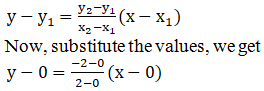y = – x

The equation of lineis y = -x

(ii) (a, b) and (a + c sinα, b + c cos α)

Given:

(x1, y1)= (a, b), (x2, y2) = (a + c sin α, b + c cos α)

So, the equation ofthe line passing through the two points (0, 0) and (2, −2) is

By using the formula,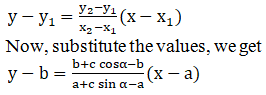y – b = cot α (x – a)

The equation of lineis y – b = cot α (x – a)

Question 2 :
Find the equations to the sides of the triangles the coordinates of whose angular points are respectively:
(i) (1, 4), (2, -3) and (-1, -2)
(ii) (0, 1), (2, 0) and (-1, -2)

(i) (1, 4), (2, -3) and(-1, -2)

Given:

Points A (1, 4), B (2,-3) and C (-1, -2).

Let us assume,

m1, m2, andm3 be the slope of the sides AB, BC and CA, respectively.

So,

The equation of theline passing through the two points (x1, y1)and (x2, y2).

Then,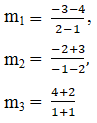m1 = -7, m2 =-1/3 and m3 = 3

So, the equation ofthe sides AB, BC and CA are

By using the formula,

y – y1= m(x – x1)

=> y – 4 = -7 (x –1)

y – 4 = -7x + 7

y + 7x = 11,

=> y + 3 = (-1/3)(x – 2)

3y + 9 = -x + 2

3y + x = – 7

x + 3y + 7 = 0 and

=> y + 2 = 3(x+1)

y + 2 = 3x + 3

y – 3x = 1

So, we get

y + 7x =11, x+ 3y + 7=0 and y – 3x = 1

The equation of sidesare y + 7x =11, x+ 3y + 7 =0 and y – 3x = 1

(ii) (0, 1), (2, 0) and(-1, -2)

Given:

Points A (0, 1), B (2,0) and C (-1, -2).

Let us assume,

m1, m2, andm3 be the slope of the sides AB, BC and CA, respectively.

So,

The equation of theline passing through the two points (x1, y1)and (x2, y2).

Then,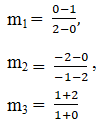m1 =-1/2, m2 = -2/3 and m3= 3

So, the equation ofthe sides AB, BC and CA are

By using the formula,

y – y1= m(x – x1)

=> y – 1 = (-1/2)(x – 0)

2y – 2 = -x

x + 2y = 2

=> y – 0 = (-2/3)(x – 2)

3y = -2x + 4

2x – 3y = 4

=> y + 2 = 3(x+1)

y + 2 = 3x + 3

y – 3x = 1

So, we get

x + 2y = 2, 2x – 3y =4and y – 3x = 1

The equation of sidesare x + 2y = 2, 2x – 3y =4 and y – 3x = 1

Question 3 : Find the equations of the medians of a triangle, the coordinates of whose vertices are (-1, 6), (-3,-9) and (5, -8).

Given:

A (−1, 6), B (−3, −9)and C (5, −8) be the coordinates of the given triangle.

Let us assume: D, E,and F be midpoints of BC, CA and AB, respectively. So, the coordinates of D, Eand F are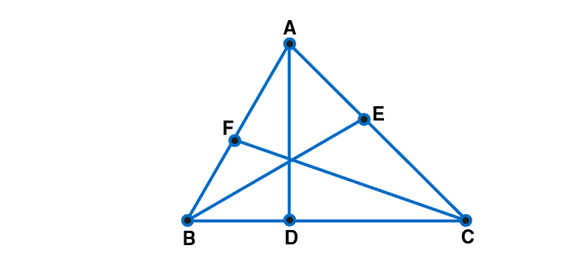Median AD passesthrough A (-1, 6) and D (1, -17/2)

So, by using theformula,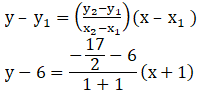4y – 24 = -29x – 29

29x + 4y + 5 = 0

Similarly, Median BEpasses through B (-3,-9) and E (2,-1)

So, by using theformula,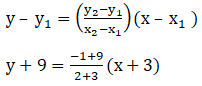5y + 45 = 8x + 24

8x – 5y – 21=0

Similarly, Median CFpasses through C (5,-8) and F(-2,-3/2)

So, by using theformula,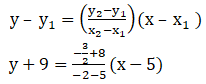-14y – 112 = 13x – 65

13x + 14y + 47 = 0

The equation of linesare: 29x + 4y + 5 = 0, 8x – 5y – 21=0 and 13x + 14y + 47 = 0

Question 4 : Find the equations to the diagonals of the rectangle the equations of whose sides are x = a, x = a’, y = b and y = b’.

Given:

The rectangle formedby the lines x = a, x = a’, y = b and y = b’

It is clear that, thevertices of the rectangle are A (a, b), B (a’, b), C (a’, b’) and D (a, b’) .

The diagonal passingthrough A (a, b) and C (a’, b’) is

By using the formula,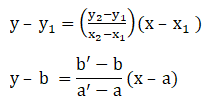(a’ – a)y – b(a’ – a)= (b’ – b)x – a(b’ – b)

(a’ – a) – (b’ – b)x =ba’ – ab’

Similarly, thediagonal passing through B (a’, b) and D (a, b’) is

By using the formula,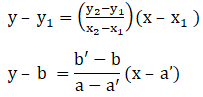(a’ – a)y – b(a – a’)= (b’ – b)x – a’ (b’ – b)

(a’ – a) + (b’ – b)x =a’b’ – ab

The equation ofdiagonals are y(a’ – a) – x(b’ – b) = a’b – ab’ and

y(a’ – a) + x(b’ – b)= a’b’ – ab

Question 5 : Find the equation of the side BC of the triangle ABC whose vertices are A (-1, -2), B (0, 1) and C (2, 0) respectively. Also, find the equation of the median through A (-1, -2).

Given:

The vertices oftriangle ABC are A (-1, -2), B(0, 1) and C(2, 0).

Let us find theequation of median through A.

So, the equation of BCis

By using the formula,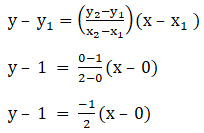x + 2y – 2 = 0

Let D be the midpointof median AD,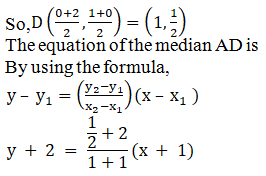4y + 8 = 5x + 5

5x – 4y – 3 = 0

The equation of lineBC is x + 2y – 2 = 0

The equation of medianis 5x – 4y – 3 = 0

Todays Deals### RD Chapter 23- The Straight Lines Ex-23.5 Contributorskrishan

Name:
Email:

# Latest News# 9000 interview questions in different categories